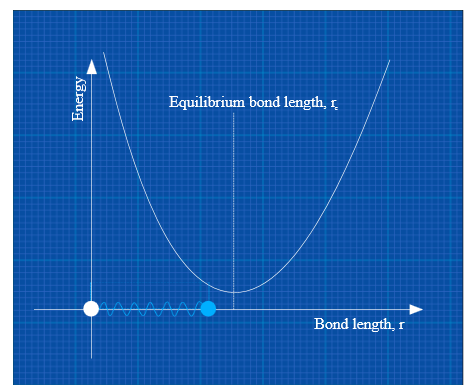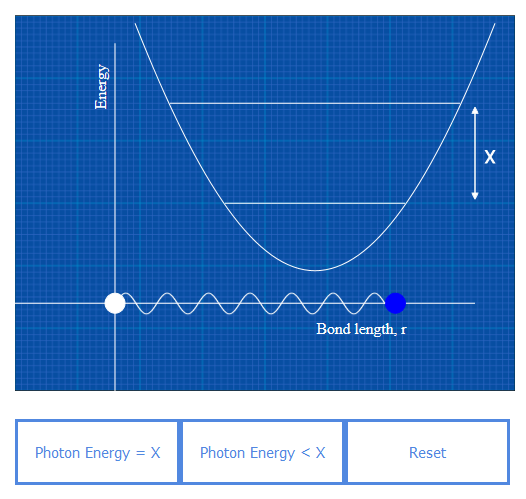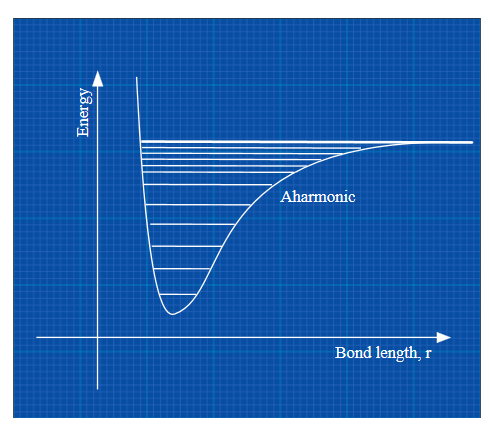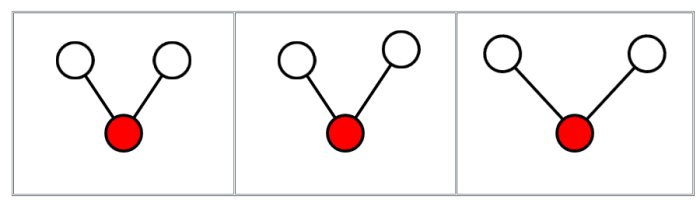What are you looking for?Molecular Vibrations Explained | Animated Guides

Courtesy of Specac Limited

How do molecules vibrate? What is anharmonicity? Read this animated guide to molecular vibration.

A basic model of molecular vibration

In this section to keep things simple we will consider the smallest possible molecule consisting of two atoms (called a diatomic molecule). On a basic level, molecules can be modelled as simple harmonic oscillators (don’t worry about this terminology just think of it as the name for this model). In this model (shown in Figure 1) we can think of the atoms as positively charged balls and the bonds between the atoms as springs holding the balls together.Figure 1: Simple harmonic oscillator model for a diatomic molecule.

As the distance between the two atoms (r) is decreased from the equilibrium bond length (re), repulsion between the positive nuclei dominates and the energy of the molecule increases, approaching infinity as the distance r approaches zero.

When r is increased beyond rthen the spring acts to pull the two balls back together and as r increases further and further the energy again tends towards infinity. Between these two extremes the repulsive and attractive forces cancel each other out forming an energy minimum and creating an energy well.

All molecules above absolute zero temperature vibrate, moving back and forth around the bottom of the energy well. When the molecule vibrates with the smallest possible maximum displacement from r(i.e. where the green ball moves the smallest distance that it can from re before returning to re) it is known as being in its ground state. When a molecule in its ground state absorbs a photon (energy from light) it will begin to vibrate more and move further from re.

As a direct consequence of quantum mechanics molecules can only have discreet quantities of energy, that is to say molecules cannot take any value of energy on the y axis and can only exist at discrete points along it. This is known as quantisation of energy.  As a result of this molecules cannot absorb just any old photon, only those that correspond to the energy gaps between the different energy levels (Figure 2). For vibrational energy levels the gaps between the levels correspond to energies in the infrared region of light.Figure 2: Simple harmonic oscillator model for a diatomic molecule showing the quantised energy levels and the effect of the interaction of the molecules with photons of light with different energies.

Infrared spectroscopy allows us to see the change in energy required to excite the molecule from the ground state into the first excited state (which we call the fundamental modes) and beyond (transitions to the second or third or forth etc.

Excited states are known as overtones). The exact spacing between the different energy levels (labelled as x in figure 2) varies depending on the size of the ball and the strength of the spring in our model (mass of the atoms and strength of the bond). As a result, different types of molecules have different IR spectra, and these spectra provide a unique finger print to identify the molecules present. The x axis of the IR spectrum is a measure of the energy of the photon and typically presented in wavenumbers / cm-1, whilst the y axis represents how much of the light is absorbed. Typically, either %transmission or absorbance is used for this axis.

Introducing anharmonicity

So far our simple model assumes that the spring can be stretched further and further forever without breaking. In the real world, eventually you will get to a point where the spring snaps. A more complex model that provides a better representation of reality is the anharmonic oscillator model as shown in Figure 3. The critical difference is that at a particular point on the x axis of the graph the energy of the molecule reaches a maximum at the point where the spring breaks and then moving the two balls further apart results in no further energy gain. The second major difference is that as a result of anharmonicity the gaps between the energy levels decreases as you get closer to bond dissociation.

In the harmonic oscillator model going from the ground to the second excited state required exactly double the energy as going from the ground to first excited state. Going from the ground  state to the third excited state required 3 times as much energy as going from the ground to the first. Once anharmonicity is introduced this is no longer the case; If the difference between going from the ground to first excited state is x then going from the ground to the second excite state will be slightly less than 2x.Figure 3 – show the harmonic model morphing into anharmonic, then show bond dissociation.

To use a real world example of hydrogen chloride gas has a fundamental IR mode at 2886 cm-1. The harmonic model would therefore predict the first overtone band (corresponding to an excitation from the ground to the second excited state) at 5772 cm-1(2x2886). However, when the spectrum of HCl is measured we find that this overtone band is at 5668 cm-1, fractionally less (4 cm-1) than that predicted by the harmonic model. Likewise, the second overtone band (Corresponding to the ground to third excited state) is at 8348 cm-1, 11 cm-1less than predicted by the harmonic oscillator model. Note: The exact spectrum of this compound is actually more complex due to the fact that it is a gas, however this is beyond the scope of the current article and will be covered in another primer at a later date.

Beyond diatomic molecules

So far we have only considered molecules consisting of 2 atoms which will show at most 1 fundamental IR band. When we begin to add more atoms the number of possible vibrations increases and more fundamental bands are observed. The total number of possible vibrations for a molecule is equal to 3N-6 (3N-5 for a linear molecule) where N is equal to the number atoms in the molecule. However, not all of these vibrations will be IR active.

To return to our example of water given above this is a bent 3 atom molecule, and from 3N-6 we predict 3x3-6=3 fundamental modes. These modes are shown in Figure 4 and correspond to: a symmetric stretch (where the two bonds grow and shrink together); an asymmetric stretch (where one bond is stretching whilst the other shrinks); and a bend where the two bonds vibrate towards and away from each other.Figure 4: Animation showing the three vibrational modes of water: (i) symmetric stretch, (ii) asymmetric stretch and (iii) bending modes.

These 3 modes are all IR active and therefore have corresponding peaks in the IR spectrum at 3490, 3247 and 1640 cm-1 (corresponding to the asymmetric stretching, symmetric stretching and bending modes). The asymmetric and symmetric modes are both relatively broad in terms of peak width and therefore overlap, however the asymmetric band is visible as a shoulder on the symmetric band as highlighted in Figure 5 which shows an example spectrum of water collected using a Specac Quest ATR.Figure 5: Infrared spectrum of water showing the 3 fundamental modes.

Get in touch: Collaborate with Specac

Are you working in a field that uses, or could use, our FTIR, XRF, polarizer, heated platen or hydraulic pressing equipment? Do you want your work reported to a wider audience? Specac collaborative research is often shared internationally via various promotional channels.